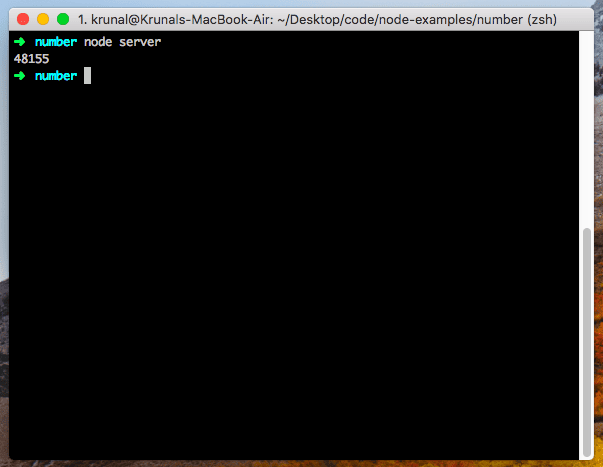# How to Convert String to Number in JavaScript

JavaScript string is a primitive data type that is immutable. JavaScript numbers can be positive or negative integers. However, integers are floating-point values.

## JavaScript string to number

To convert a string to a number in JavaScript, use the parseInt() function. Javascript parseInt() is a built-in function that converts a string to a number(integer).

Create a file called node.js and add the following code.

```const data = Number('48155');

console.log(data);```

Go to the terminal and start the node.js server.

`node server`

It will also take care of the decimal as well.

A Number is a wrapper object that can perform many operations. If we use the constructor (`new Number("48155")`) it returns us a Number object instead of a number value, so pay attention.## JavaScript parseInt()

The parseInt() method converts a string into an integer (a whole number). It accepts two arguments. The first argument is the string to convert.

```// server.js

let text = '21px';
let integer = parseInt(text, 10);

console.log(integer);```

It will log the 21 value.

## Javascript parseFloat()

The parseFloat() is a built-in JavaScript method that converts a string into a point number (a number with decimal points).

```// server.js

let text = '3.14pie';
let integer = parseFloat(text, 10);

console.log(integer);```

## + Unary Operator

One “trick” is to use the unary operator + before the string. The unary plus is used when your string is already in the form of an integer.

```// server.js

let x = 5+"1000";

console.log(x);```

The output will be 51000.

## Math.floor()

The Math.floor() is a built-in JavaScript function that rounds down and returns the largest integer less than or equal to a given number. It is the + unary operator but returns the integer part.

```// server.js

let floor = Math.floor("1000.51");
console.log(floor);```